BRAIN TEASERSpuzzles, riddles, mathematical problems, mastermind, cinemania... These are the tasks listed 1 to 10.

## MAGIC SQUARE: Calculate A-B+C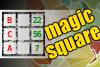The aim is to place the some numbers from the list (7, 9, 14, 15, 16, 22, 54, 56, 62, 86) into the empty squares and squares marked with A, B an C. Sum of each row and column should be equal. All the numbers of the magic square must be different. Find values for A, B, and C. Solution is A-B+C.
#brainteasers #math #magicsquare

## Calculate the number 1366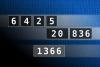NUMBERMANIA: Calculate the number 1366 using numbers [6, 4, 2, 5, 20, 836] and basic arithmetic operations (+, -, *, /). Each of the numbers can be used only once.
#brainteasers #math #numbermania

## Calculate the number 2182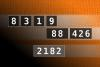NUMBERMANIA: Calculate the number 2182 using numbers [8, 3, 1, 9, 88, 426] and basic arithmetic operations (+, -, *, /). Each of the numbers can be used only once.
#brainteasers #math #numbermania### Toughest job I ever had

Toughest job I ever had: selling doors, door to door.

Bill Bailey (January 13 1964-)

Jokes of the day - Daily updated jokes. New jokes every day.

## Calculate the number 2501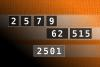NUMBERMANIA: Calculate the number 2501 using numbers [2, 5, 7, 9, 62, 515] and basic arithmetic operations (+, -, *, /). Each of the numbers can be used only once.
#brainteasers #math #numbermania

## Find number abc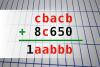If cbacb + 8c650 = 1aabbb find number abc. Multiple solutions may exist.
#brainteasers #math

## Find number abc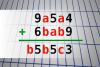If 9a5a4 + 6bab9 = b5b5c3 find number abc. Multiple solutions may exist.
#brainteasers #math

## MAGIC SQUARE: Calculate A+B*C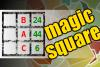The aim is to place the some numbers from the list (6, 7, 13, 17, 18, 24, 43, 44, 50, 72) into the empty squares and squares marked with A, B an C. Sum of each row and column should be equal. All the numbers of the magic square must be different. Find values for A, B, and C. Solution is A+B*C.
#brainteasers #math #magicsquare

## Calculate the number 2901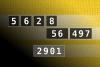NUMBERMANIA: Calculate the number 2901 using numbers [5, 6, 2, 8, 56, 497] and basic arithmetic operations (+, -, *, /). Each of the numbers can be used only once.
#brainteasers #math #numbermania

## Calculate the number 8175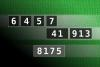NUMBERMANIA: Calculate the number 8175 using numbers [6, 4, 5, 7, 41, 913] and basic arithmetic operations (+, -, *, /). Each of the numbers can be used only once.
#brainteasers #math #numbermania

## MAGIC SQUARE: Calculate A-B-C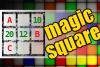The aim is to place the some numbers from the list (9, 10, 12, 19, 20, 22, 38, 70, 71, 73, 86) into the empty squares and squares marked with A, B an C. Sum of each row and column should be equal. All the numbers of the magic square must be different. Find values for A, B, and C. Solution is A-B-C.
#brainteasers #math #magicsquare
 NEXT PAGE
 Follow Brain Teasers on social networks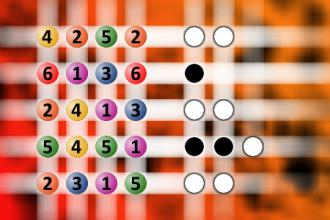The computer chose a secret code (sequence of 4 digits from 1 to 6). Your goal is to find that code. Black circles indicate the number of hits on the right spot. White circles indicate the number of hits on the wrong spot.

## Top 10 Users (1174)

 1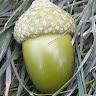H Tav 5861 2c. raj. 5777 3Nasrin 24 T 5731 4Fazil Hashim 5718 5Thinh Ddh 5557 6Alfa Omega 4681 7Djordje Timotijevic 4549 8Mita Kojd 4525 9Jakubovski Vladimir 4443 10Chandu Rajyaguru 4419
See full ranking list